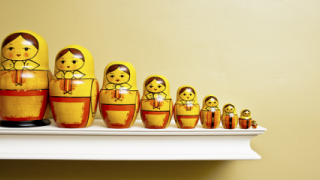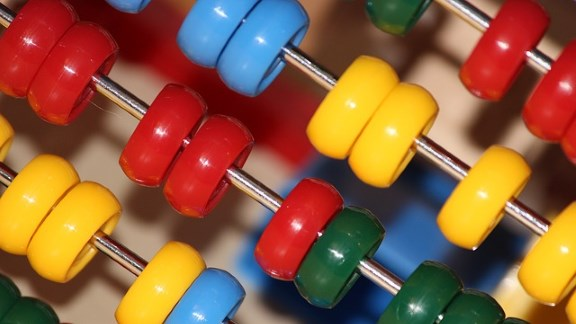# Exploring Our Number System

Simple ideas, like adding together two odds to make an even, can lead to fascinating insights with important real world applications.We offer you some simple starting points exploring factors and multiples, remainders and number bases, the foundations of the field mathematicians call Number Theory.### Factors and Multiples Chain

Each number must be a factor or multiple of the last. What's the longest chain you can make?### Weights

Imagine you had a set of positive and negative "weights", which can be combined to make different totals. Which totals can you make?### Remainders

When we divide, we're rarely interested in what's left over. In these problems and games, we invite you to take a closer look at remainders and learn about Modular Arithmetic, an important area of Number Theory.### Factors and Multiples

Factors and multiples are the building blocks of Number Theory. Try these problems and games and get to grips with the underlying structure of our number system.### Number Bases

We count in base ten because most of us have ten fingers. But what if we only had seven or eight? Here's a chance for you to explore the properties of base 10 as well as some less familiar number bases.